# Selina Solutions Concise Maths Class 7 Chapter 17: Symmetry (Including Reflection and Rotation) Exercise 17B

Selina Solutions Concise Maths Class 7 Chapter 17 Symmetry (Including Reflection and Rotation) Exercise 17B improves problem solving skills among students. Reflection along x-axis, y-axis and origin is the topic explained under this exercise. The solved examples and stepwise solutions help students in solving difficult problems with ease. The solutions PDF can be referred by students while solving the textbook problems, without any time constraints. Students can avail Selina Solutions Concise Maths Class 7 Chapter 17 Symmetry (Including Reflection and Rotation) Exercise 17B PDF, from the links which are given here.

## Selina Solutions Concise Maths Class 7 Chapter 17: Symmetry (Including Reflection and Rotation) Exercise 17B Download PDF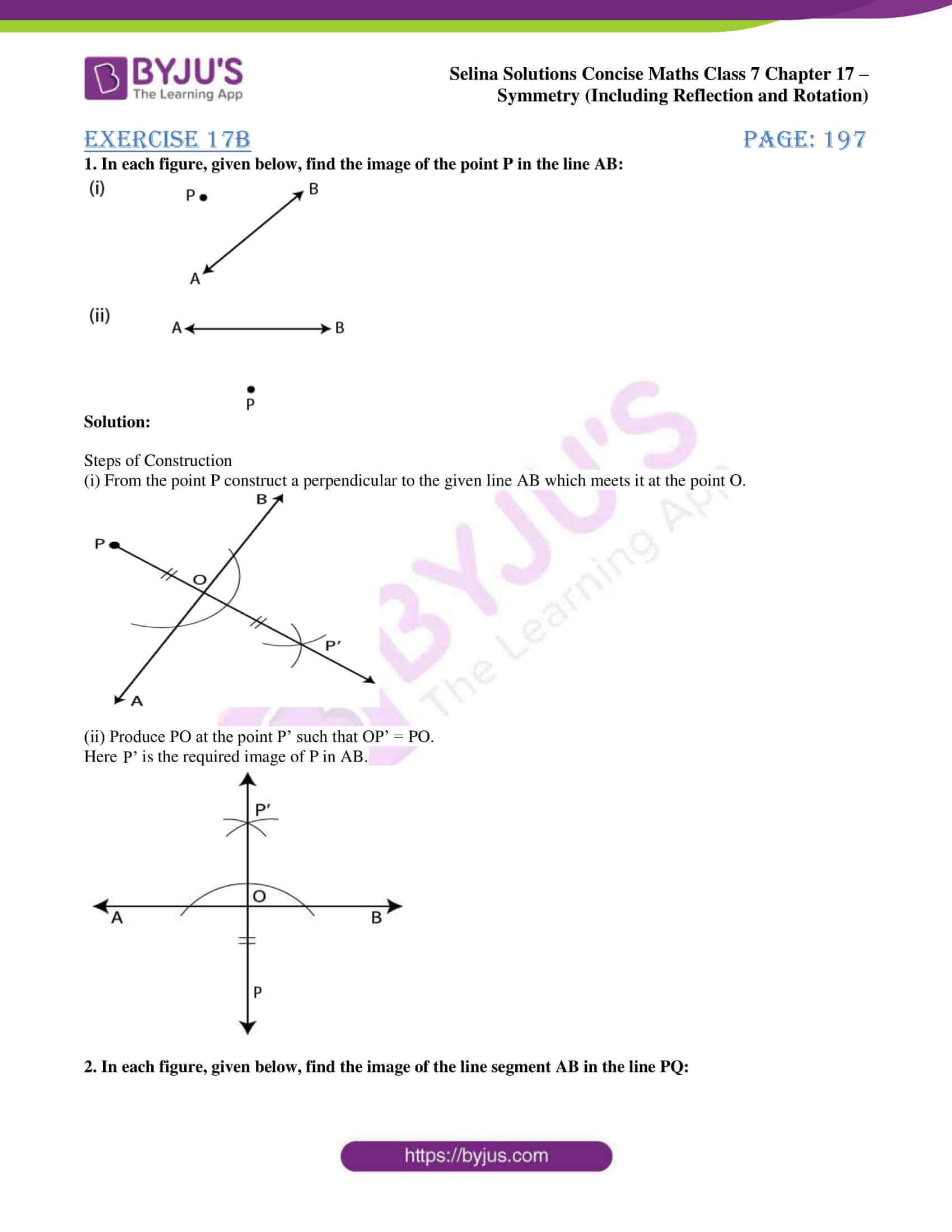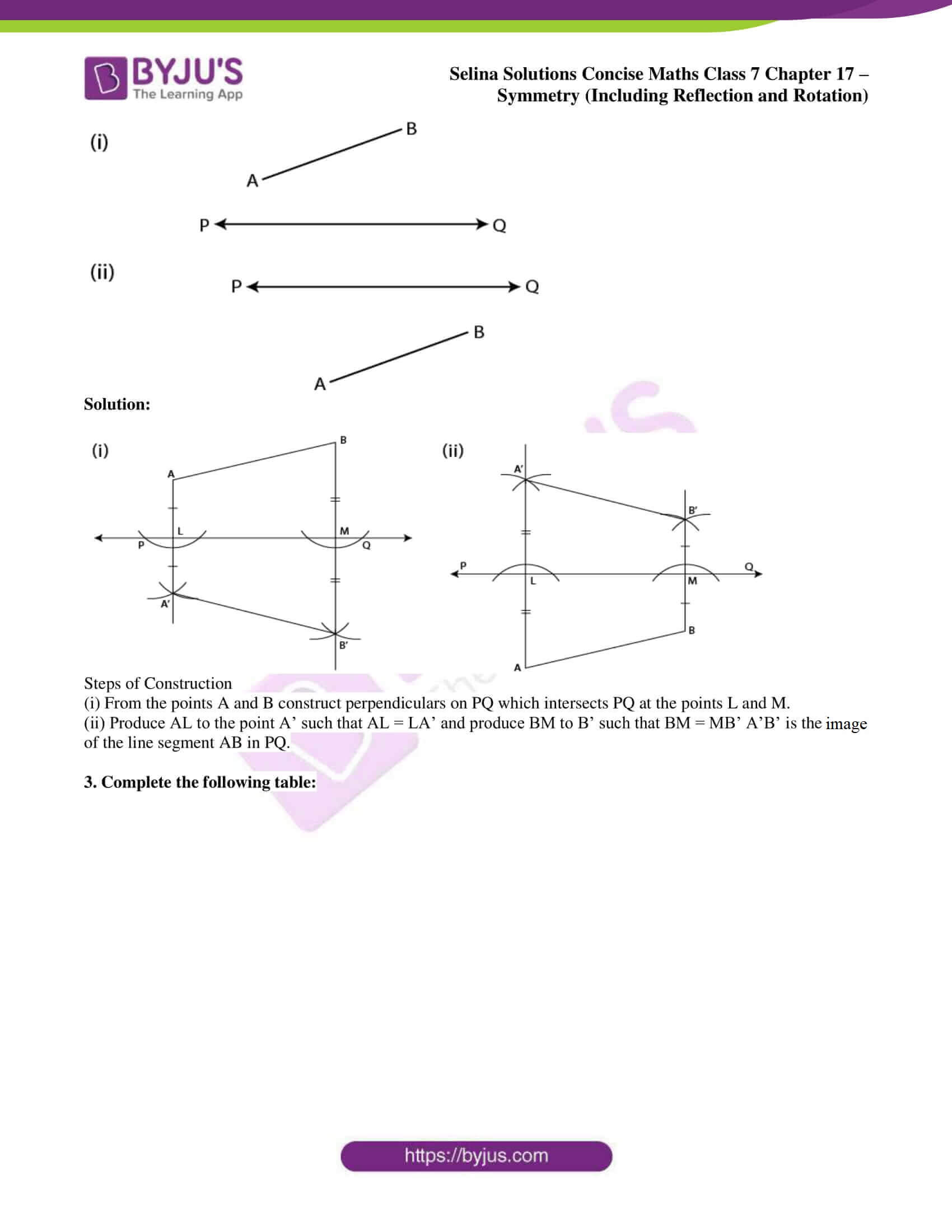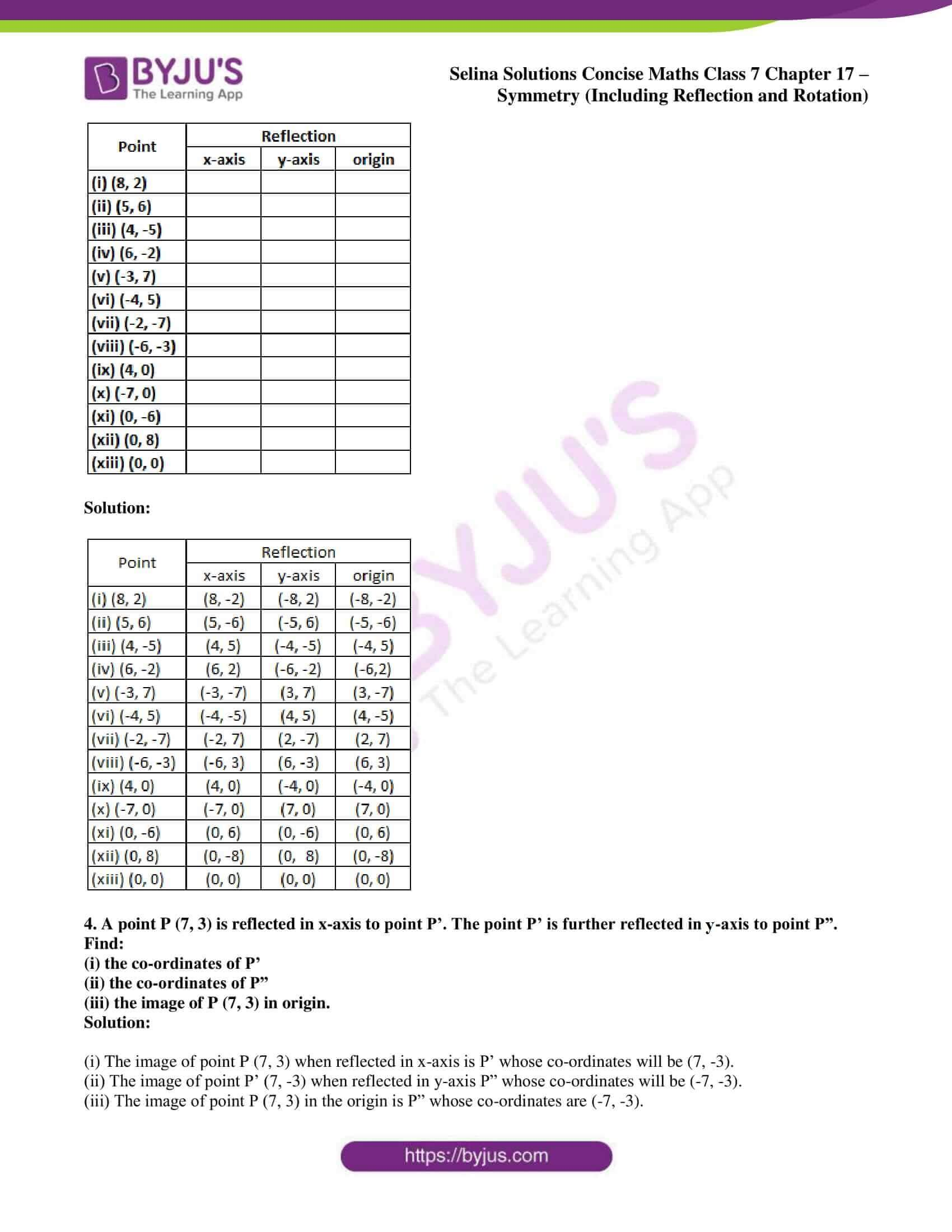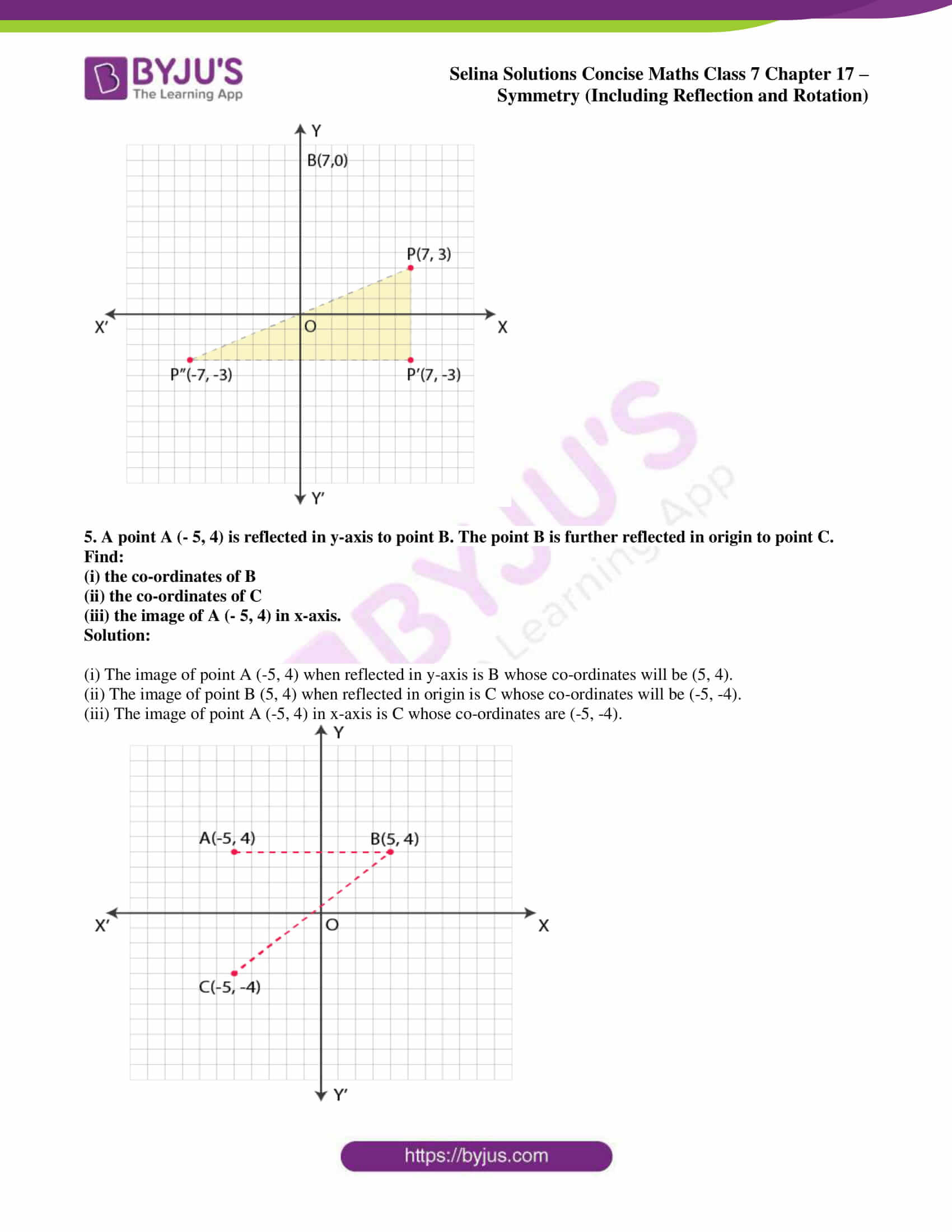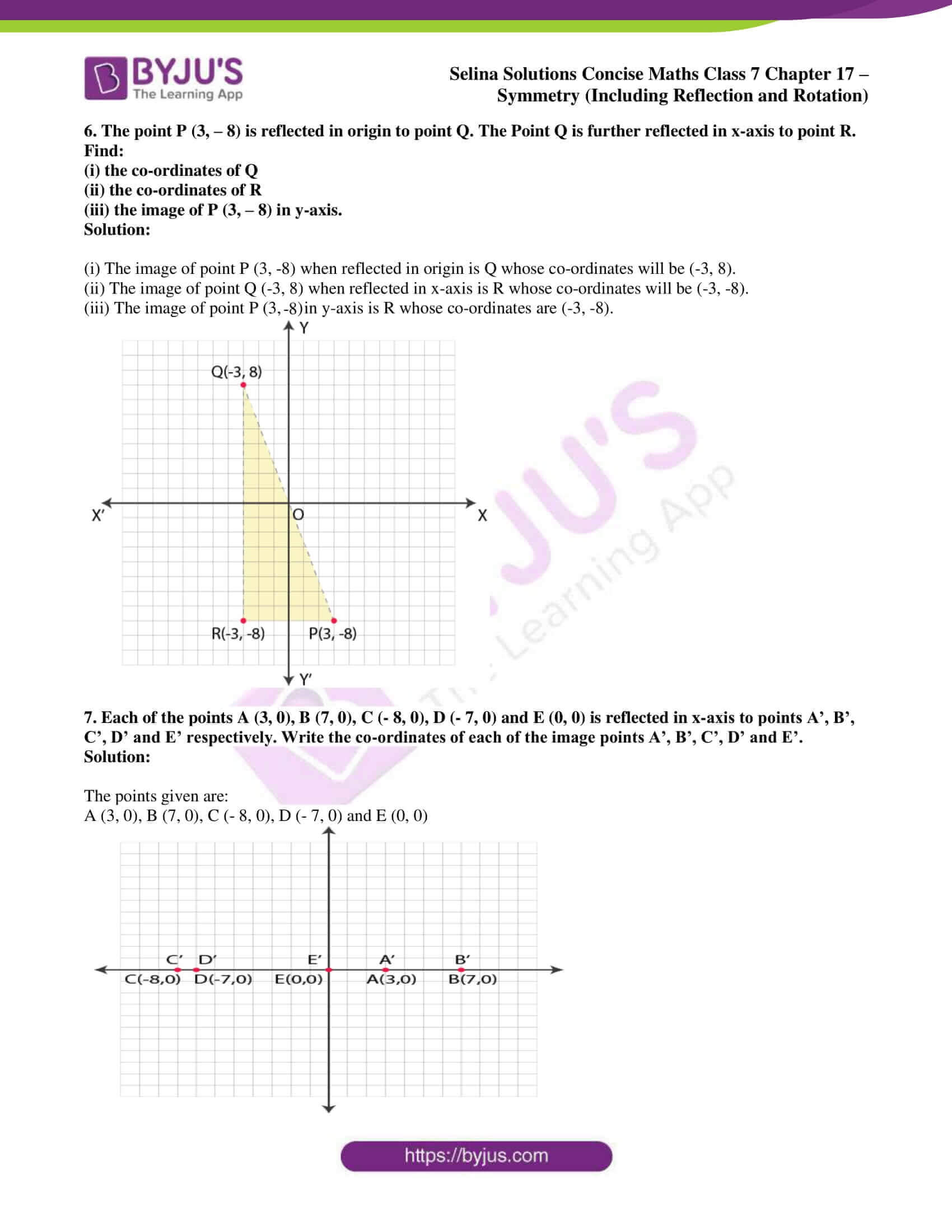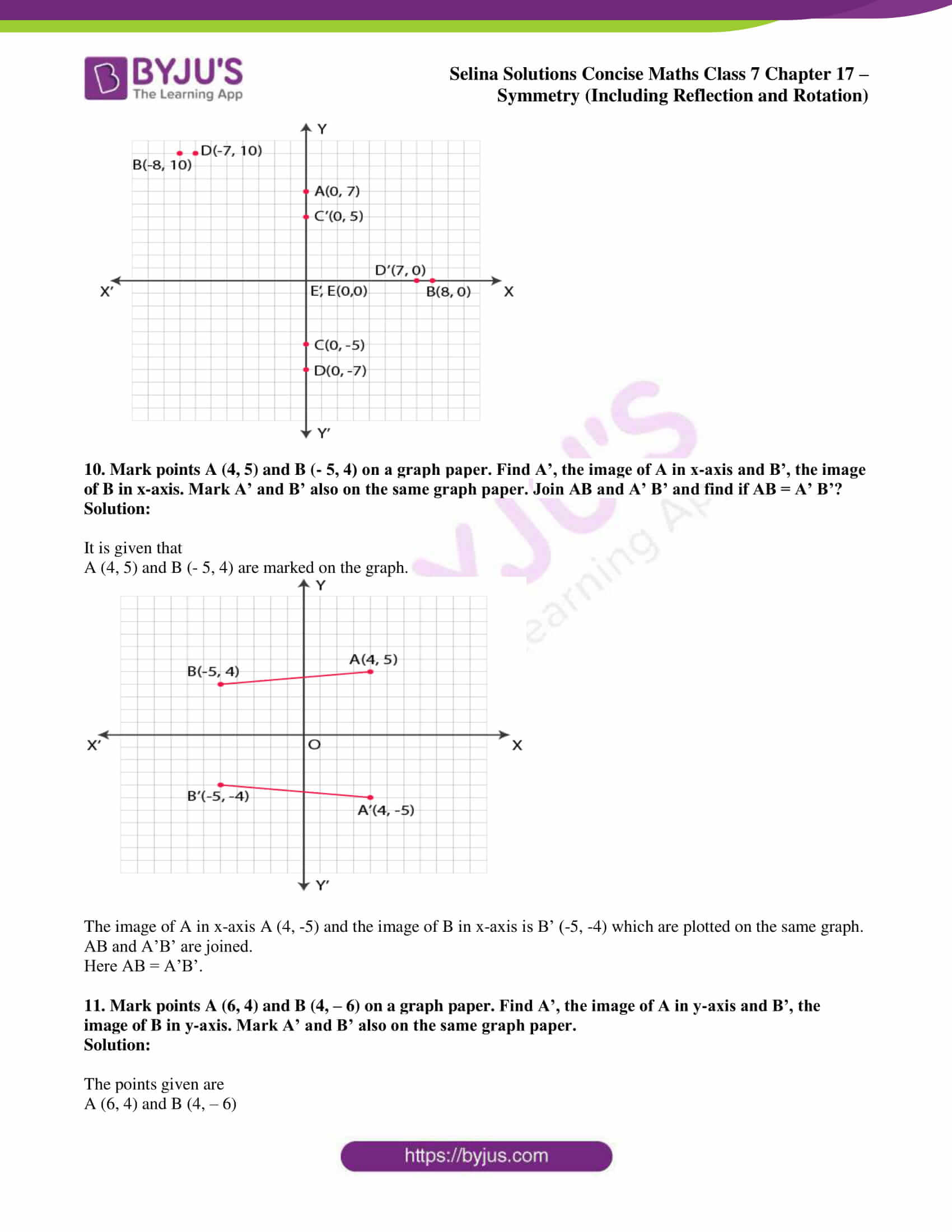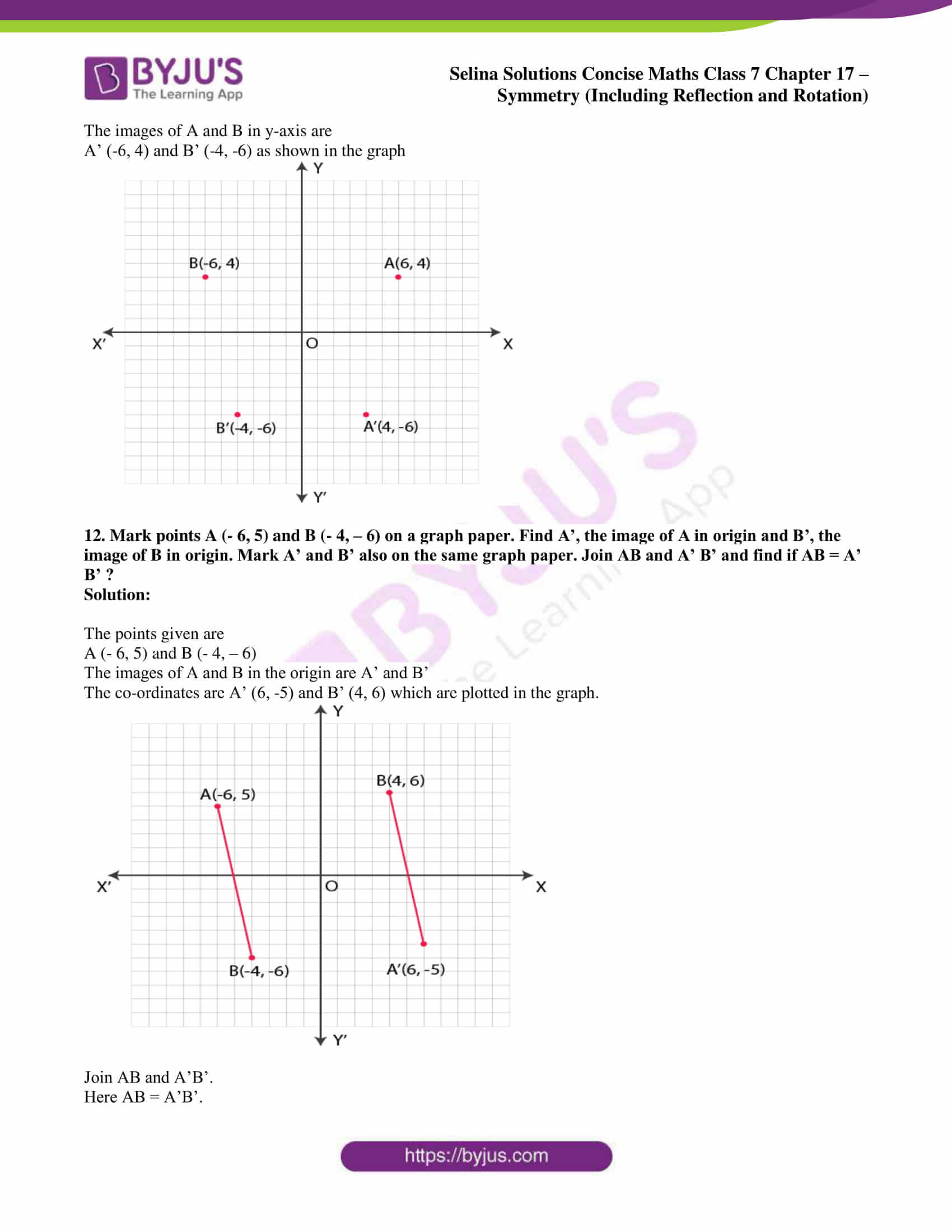### Access other exercises of Selina Solutions Concise Maths Class 7 Chapter 17: Symmetry (Including Reflection and Rotation)

Exercise 17A Solutions

Exercise 17C Solutions

### Access Selina Solutions Concise Maths Class 7 Chapter 17: Symmetry (Including Reflection and Rotation) Exercise 17B

#### Exercise 17B PAGE: 197

1. In each figure, given below, find the image of the point P in the line AB: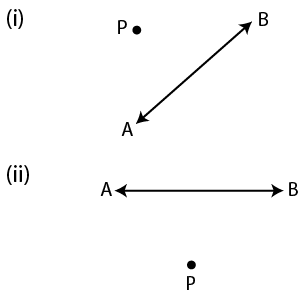Solution:

Steps of Construction

(i) From the point P construct a perpendicular to the given line AB which meets it at the point O.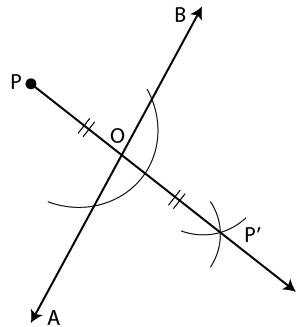(ii) Produce PO at the point P’ such that OP’ = PO.

Here P’ is the required image of P in AB.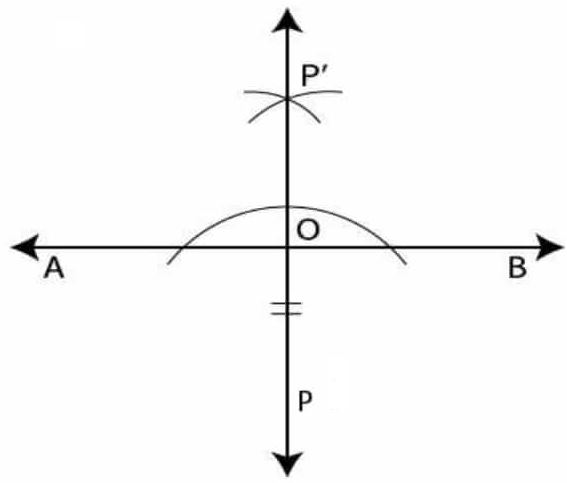2. In each figure, given below, find the image of the line segment AB in the line PQ: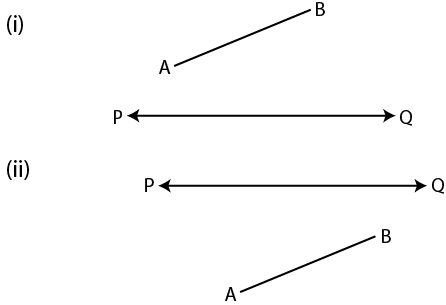Solution: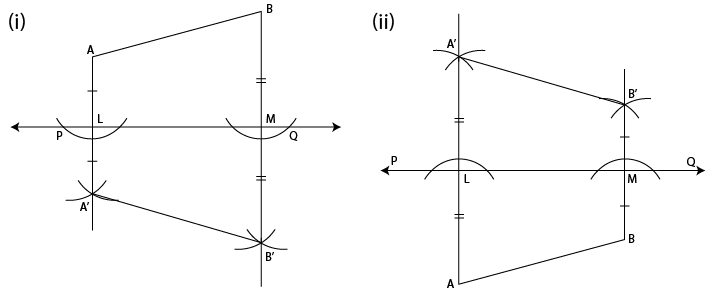Steps of Construction

(i) From the points A and B construct perpendiculars on PQ which intersects PQ at the points L and M.

(ii) Produce AL to the point A’ such that AL = LA’ and produce BM to B’ such that BM = MB’ A’B’ is the image of the line segment AB in PQ.

3. Complete the following table: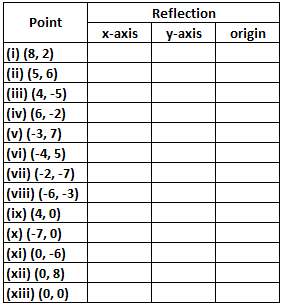Solution: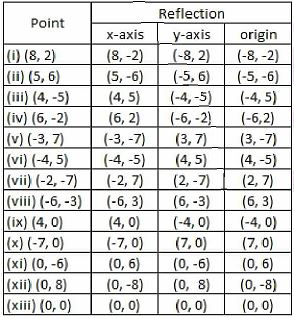4. A point P (7, 3) is reflected in x-axis to point P’. The point P’ is further reflected in y-axis to point P”. Find:

(i) the co-ordinates of P’

(ii) the co-ordinates of P”

(iii) the image of P (7, 3) in origin.

Solution:

(i) The image of point P (7, 3) when reflected in x-axis is P’ whose co-ordinates will be (7, -3).

(ii) The image of point P’ (7, -3) when reflected in y-axis P” whose co-ordinates will be (-7, -3).

(iii) The image of point P (7, 3) in the origin is P” whose co-ordinates are (-7, -3).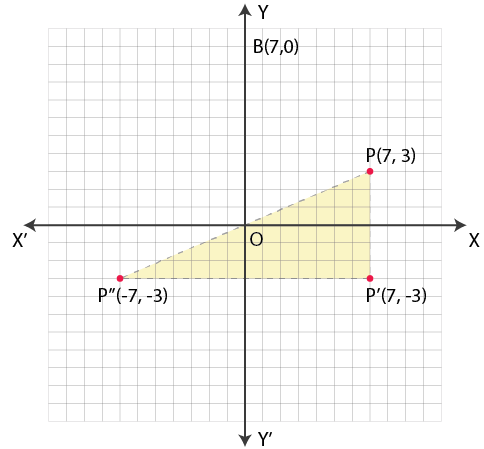5. A point A (- 5, 4) is reflected in y-axis to point B. The point B is further reflected in origin to point C. Find:

(i) the co-ordinates of B

(ii) the co-ordinates of C

(iii) the image of A (- 5, 4) in x-axis.

Solution:

(i) The image of point A (-5, 4) when reflected in y-axis is B whose co-ordinates will be (5, 4).

(ii) The image of point B (5, 4) when reflected in origin is C whose co-ordinates will be (-5, -4).

(iii) The image of point A (-5, 4) in x-axis is C whose co-ordinates are (-5, -4).6. The point P (3, – 8) is reflected in origin to point Q. The Point Q is further reflected in x-axis to point R. Find:

(i) the co-ordinates of Q

(ii) the co-ordinates of R

(iii) the image of P (3, – 8) in y-axis.

Solution:

(i) The image of point P (3, -8) when reflected in origin is Q whose co-ordinates will be (-3, 8).

(ii) The image of point Q (-3, 8) when reflected in x-axis is R whose co-ordinates will be (-3, -8).

(iii) The image of point P (3, -8) in y-axis is R whose co-ordinates are (-3, -8).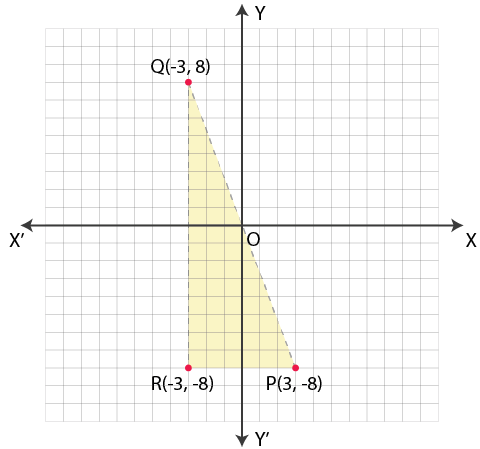7. Each of the points A (3, 0), B (7, 0), C (- 8, 0), D (- 7, 0) and E (0, 0) is reflected in x-axis to points A’, B’, C’, D’ and E’ respectively. Write the co-ordinates of each of the image points A’, B’, C’, D’ and E’.

Solution:

The points given are:

A (3, 0), B (7, 0), C (- 8, 0), D (- 7, 0) and E (0, 0)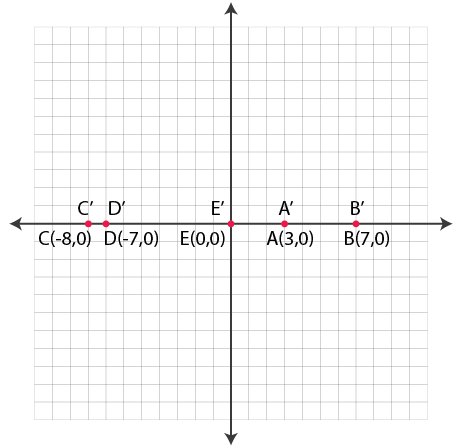The images will be reflected in x-axis

A’ (3, 0), B’ (7, 0), C’ (-8, 0), D’ (-7, 0) and E’ (0, 0) as the points lie on x-axis.

8. Each of the points A (0, 4), B (0, 10), C (0, – 4), D (0, – 6) and E (0, 0) is reflected in y-axis to points A’, B’, C’, D’ and E’ respectively. Write the co-ordinates of each of the image points A’, B’, C’, D’ and E’.

Solution:

The points given are

A (0, 4), B (0, 10), C (0, – 4), D (0, – 6) and E (0, 0) which are reflected on y-axis

The co-ordinates of their images will be

A’ (0, 4), B’ (0, 10), C’ (0, -4), D’ (0, -6) and E’ (0, 0) as they lie on y-axis.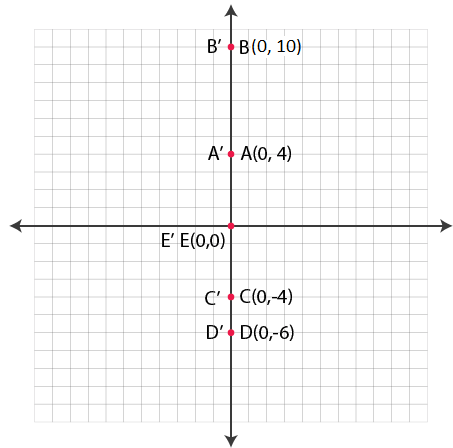9. Each of the points A (0, 7), B (8, 0), C (0, -5), D (- 7, 0) and E (0, 0) are reflected in origin to points A’, B’, C’, D’ and E’ respectively. Write the co-ordinates of each of the image points A’, B’, C’, D’ and E’.

Solution:

It is given that

A (0, 7), B (8, 0), C (0, -5), D (- 7, 0) and E (0, 0) are reflected in origin

The co-ordinates of their images will be

A’ (0, -7), B’ (-8, 0), C’ (0, 5), D’ (7, 0) and E’ (0, 0)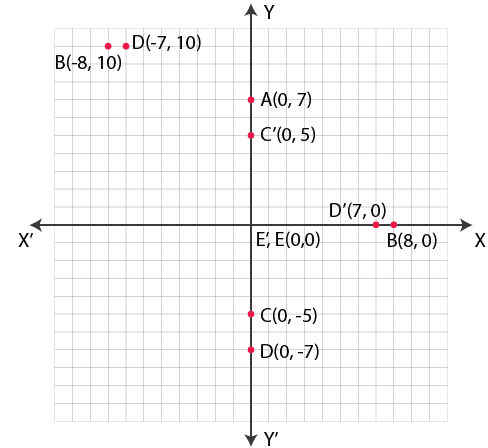10. Mark points A (4, 5) and B (- 5, 4) on a graph paper. Find A’, the image of A in x-axis and B’, the image of B in x-axis. Mark A’ and B’ also on the same graph paper. Join AB and A’ B’ and find if AB = A’ B’?

Solution:

It is given that

A (4, 5) and B (- 5, 4) are marked on the graph.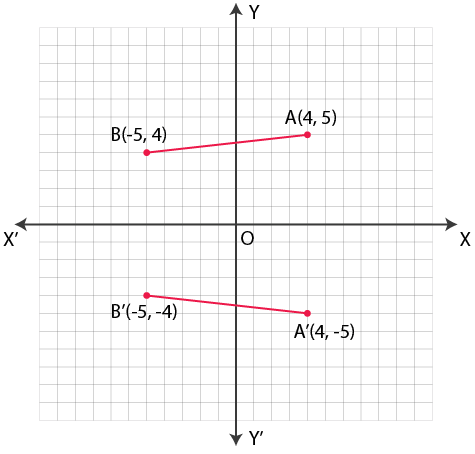The image of A in x-axis A (4, -5) and the image of B in x-axis is B’ (-5, -4) which are plotted on the same graph.

AB and A’B’ are joined.

Here AB = A’B’.

11. Mark points A (6, 4) and B (4, – 6) on a graph paper. Find A’, the image of A in y-axis and B’, the image of B in y-axis. Mark A’ and B’ also on the same graph paper.

Solution:

The points given are

A (6, 4) and B (4, – 6)

The images of A and B in y-axis are

A’ (-6, 4) and B’ (-4, -6) as shown in the graph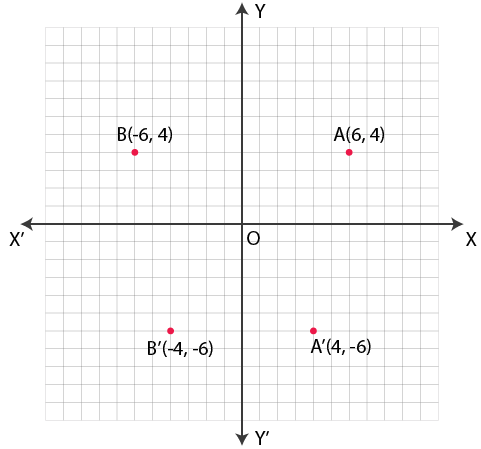12. Mark points A (- 6, 5) and B (- 4, – 6) on a graph paper. Find A’, the image of A in origin and B’, the image of B in origin. Mark A’ and B’ also on the same graph paper. Join AB and A’ B’ and find if AB = A’ B’ ?

Solution:

The points given are

A (- 6, 5) and B (- 4, – 6)

The images of A and B in the origin are A’ and B’

The co-ordinates are A’ (6, -5) and B’ (4, 6) which are plotted in the graph.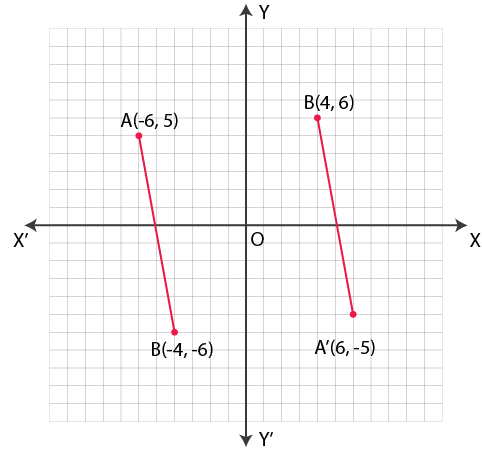Join AB and A’B’.

Here AB = A’B’.